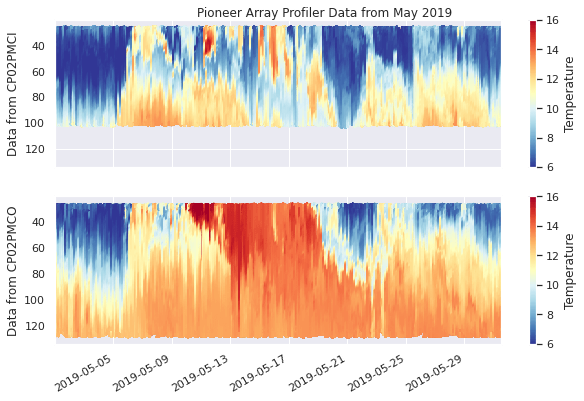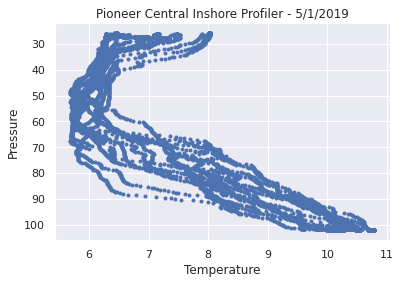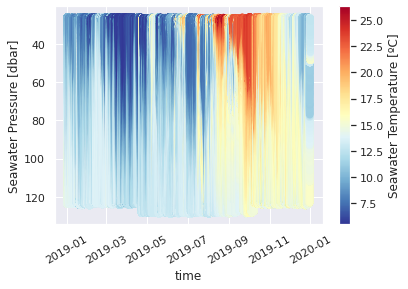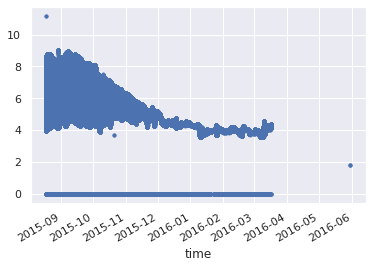# Data Labs Tutorial at WHOIToday I had the opportunity to virtually present an introduction to the Ocean Data Labs project, along with a short tutorial on working with OOI Profiler data to the WHOI Ocean Informatics Working Group. We had over 40 participants attend, including undergraduate students, faculty and career scientists.(Oh, and Tropical Storm Isaias was barreling down on me here in NJ.  Luckily the power held out at my house… but if I had actually been in the office, I wouldn’t have been so lucky. Go figure.)

It was fun session to put together, though I’m sure if would have been far easier in person. I covered a lot of ground (and in retrospect, probably way too much). But the content was partly driven by the diverse audience we had. Not only did the participants range from undergrads to tenured faculty, but also from python novices to experts, as well as OOI newbies to old-hands.

Here are just a few of the topics we tried to cover…

• An introduction to the Data Labs project and our Community of Practice
• A brief overview of the power and benefits of python notebooks for reproducible research
• A nickel tour of the OOI
• Including working with THREDDS, NetCDF files, loading single vs. multiple files, and the #fillmismatch hack
• And finally, actually visualizing some fun data from the Pioneer Array Profilers

In the end we didn’t get too far through the notebook I created.  But that’s the beauty of notebooks.  All of the text, code and practice exercises I prepared are all there for participants to take a look at at their leisure.

The reality is, working with large complex datasets from observatories like the OOI, which itself is designed to measure complex processes, and using new tools (like Python) to work with them, is a lot to learn.  In a 1-hour tutorial, you can barely scratch the surface.  To really delve into these topics, you really need a full course (at a minimum) to practice all the skills that help you discover the stories hidden in the data.  One day, I’d love to lead one. If you’re interested in making this happen, please ping me.

But in an hour, you can definitely excite people about the potential of what’s possible.  And that’s always my goal.  So if you attended today, I hope you found some benefit, and are eager to learn more.

For those who are interested in diving in to what was presented today, you can check out the slides I presented (ppt, pdf), as well as the webinar recording, and the full notebook below.  If you would like to open the notebook in Colab to play with it yourself, you can use use this link.

Special thanks to Stace Beaulieu at WHOI for organizing the event and virtually hosting me!

# OOI Profile Examples - WHOI Data Tutorial¶

Written by Sage Lichtenwalner, Rutgers University, August 4, 2020

The example was developed as a Data Tutorial for the WHOI Ocean Informatics Working Group.

## Introduction¶

Working with data from the OOI presents a few challenges to a new user. But the good news is, once you figure out how to load a dataset from one instrument, which only takes a few lines of code in Python, you then have all the tools you need to load data from almost any instrument available in the OOI Data Portal.

All of the data in the Data Portal is made available in the same format (basically a 1-dimensional timeseries available as either downloadable NetCDF files or via a THREDDS server).

Thus, you can use the same code to access data from fixed instruments on moorings, underwater nodes, wire-following profilers, or even gliders. Even ADCP and OPTAA data is very similar in format (albeit with a 2nd dimension for bin or frequency), making them easy to work with as well.

That said, data from profilers and gliders present more of a challenge, because the actual data we want is in 3D (time, depth and measured variable), which requires more advanced plotting techniques. In addition, there are often other quirks in the dataset that have to be dealt with.In this Python notebook, we will demonstrate how to access and plot some profiler and glider data from the Ocean Observatories Initiative (OOI), specifically from the Pioneer Array.

This example was designed to run on Google's Colaboratory platform, though it should also work on any Jupyter notebook platform, assuming the required libraries are installed.

## 1. Requesting Data¶

As of July 2020, in order to use data from the OOI, you must first request the dataset that you are interested in, including the instrument, "method", "stream", and time range that you want.

There are two ways to do this:

• Use the OOI Data Portal - Good for exploring what's available, and when you only want to make a few requests.
• Use the API - Good for when you want to make a lot of requests (e.g. for different instruments or time ranges).

This example assumes you've already requested the data and have a "THREDDS URL" to work with. To learn more about how to make a request via the API, check out this example

To start, we will use data from the Wire-Following Profiler - CTD sensor from the following sites:

In :
```# Notebook setup
import requests
import os
import re
import xarray as xr
!pip install netcdf4
import pandas as pd
import matplotlib.pyplot as plt

# Make the Plots pretty
import seaborn as sns
sns.set()

# Supress open_mfdataset warnings
import warnings
warnings.filterwarnings('ignore')
```
```Requirement already satisfied: netcdf4 in /usr/local/lib/python3.6/dist-packages (1.5.0)
Requirement already satisfied: numpy>=1.7 in /usr/local/lib/python3.6/dist-packages (from netcdf4) (1.18.5)
Requirement already satisfied: cftime in /usr/local/lib/python3.6/dist-packages (from netcdf4) (1.2.1)
```
```/usr/local/lib/python3.6/dist-packages/statsmodels/tools/_testing.py:19: FutureWarning: pandas.util.testing is deprecated. Use the functions in the public API at pandas.testing instead.
import pandas.util.testing as tm
```

In this first example, we will load data from a single file over the OOI THREDDS Server. To get the correct URL, you will need to:

1. Go to the THREDDS Data Server link for the dataset
2. Select the file (.nc) that you want
3. Click on the OPENDAP link
4. Copy the "Data URL" and enter that in your code.
In :
```# CP02PMCI May 2019
data_url = 'https://opendap.oceanobservatories.org/thredds/dodsC/ooi/[email protected]/20190531T172721-CP02PMCI-WFP01-03-CTDPFK000-telemetered-ctdpf_ckl_wfp_instrument/deployment0012_CP02PMCI-WFP01-03-CTDPFK000-telemetered-ctdpf_ckl_wfp_instrument_20190501T000001-20190531T122348.982692.nc'
```
In :
```# Load the data files
ds = xr.open_dataset(data_url)
ds = ds.swap_dims({'obs': 'time'}) #Swap dimensions
print('Dataset has %d points' % ds.time.size)
```
```Dataset has 261990 points
```
In :
```# Your Turn - Investigate the Dataset
```
In :
```%%time
# And convert to a Pandas DataFrame - This can take a while with a lot of points
df = ds.to_dataframe()
```
```CPU times: user 1.57 s, sys: 318 ms, total: 1.89 s
Wall time: 18.6 s
```
In :
```# Your Turn - Display the first few rows of the Dataframe
```

As you can see, the dataset has a lot of columns, many of which we don't need that will take up a lot of memory.

You can save memory and processing time by selecting only the columns you need up front.

In :
```# Specify only a few columns to convert to Pandas DataFrame
df = ds[['ctdpf_ckl_seawater_pressure','ctdpf_ckl_seawater_temperature','practical_salinity','density']].to_dataframe()
```

## 3. Plotting Profiler Data¶

Now let's use the "built-in" plotting features of Pandas to quickly generate some timeseries plots.

In :
```# Timeseries Quickplot
df.ctdpf_ckl_seawater_temperature.plot();
```But what if we want more control on how we plot the data? For this, it's sometimes easier to Matplotlib's plotting functions directly, so we can specify both the x and y axes independently.

Let's use Matplotlib to plot the temperature data vs. pressure.

In :
```# Profile Plot
plt.plot(df.ctdpf_ckl_seawater_temperature,df.ctdpf_ckl_seawater_pressure, linestyle='', marker='.');

# Flip the y-axis
ax = plt.gca()
ax.invert_yaxis()

# Label the Plot
plt.ylabel('Pressure')
plt.xlabel('Temperature');
plt.title('Pioneer Central Inshore Profiler - May 2019');
```This is kind of a crazy plot, because we're plotting an entire month of temperature profiles. We could subset the data to just one day.

In :
```# Subset the dataframe
df2 = df['2019-05-01':'2019-05-01']

# Profile Plot
plt.plot(df2.ctdpf_ckl_seawater_temperature,df2.ctdpf_ckl_seawater_pressure, linestyle='', marker='.');

# Flip the y-axis
ax = plt.gca()
ax.invert_yaxis()

# Label the Plot
plt.ylabel('Pressure')
plt.xlabel('Temperature');
plt.title('Pioneer Central Inshore Profiler - 5/1/2019');
```In :
```# Your Turn - Remake the plots above with Salinity or Density
```

So that's interesting. But to get a more complete picture, let's create a profile timeseries plot, which is sometimes called a Hovmoller diagram.

This can take some time, depending on how many points you have to plot.

In :
```# Scatterplots of Temperature and Salinity
fig, (ax1,ax2) = plt.subplots(2,1, sharex=True, sharey=True, figsize=(10,6))

sc1 = ax1.scatter(df.index, df.ctdpf_ckl_seawater_pressure, c=df.ctdpf_ckl_seawater_temperature, cmap='RdYlBu_r', s=2)
sc2 = ax2.scatter(df.index, df.ctdpf_ckl_seawater_pressure, c=df.practical_salinity, cmap='Blues', s=2)

# Because the X and Y axes are shared, we only have to set limits once
ax1.invert_yaxis() # Invert y axis
ax1.set_xlim(df.index,df.index[-1]) # Set the time limits to match the dataset

cbar = fig.colorbar(sc1, ax=ax1, orientation='vertical')
cbar.ax.set_ylabel('Temperature')
cbar = fig.colorbar(sc2, ax=ax2, orientation='vertical')
cbar.ax.set_ylabel('Salinity')

ax1.set_ylabel('Pressure (dbar)')
ax2.set_ylabel('Pressure (dbar)')

fig.suptitle('Data from %s' % ds.subsite)
fig.autofmt_xdate()
```This is a very interesting dataset, and certainly not something you see in a textbook. There's a lot of cool oceanography going on in the Pioneer Array.

The above steps worked with a single file... but the data portal will often returns multiple files, especially if your request spans multiple deployments. Given that the Pioneer Array is redeployed every 6 months, this is likely.

Here is a straight-forward way to load a larger OOI dataset in Python using their THREDDS Server.

In :
```# All of 2019 for CP02PMCO
data_url = 'https://opendap.oceanobservatories.org/thredds/catalog/ooi/[email protected]/20200731T174219126Z-CP02PMCO-WFP01-03-CTDPFK000-recovered_wfp-ctdpf_ckl_wfp_instrument_recovered/catalog.html'
```
In :
```def get_datalist(url):
'''Return all the relevant .nc files from a specified OOI THREDDS url'''
tds_url = 'https://opendap.oceanobservatories.org/thredds/dodsC'
datasets = requests.get(url).text
urls = re.findall(r'href=[\'"]?([^\'" >]+)', datasets)
x = re.findall(r'(ooi/.*?.nc)', datasets)
for i in x:
if i.endswith('.nc') == False:
x.remove(i)
for i in x:
try:
float(i[-4])
except:
x.remove(i)
datasets = [os.path.join(tds_url, i) for i in x]

# Remove extraneous data files if necessary
catalog_rms = url.split('/')[-2][20:]
selected_datasets = []
for d in datasets:
if catalog_rms == d.split('/')[-1].split('_20')[15:]:
selected_datasets.append(d + '#fillmismatch') # Add #fillmismatch to the URL to deal with a bug
selected_datasets = sorted(selected_datasets)
return selected_datasets
```
In :
```# List of data files
data_files = get_datalist(data_url)
data_files
```
Out:
```['https://opendap.oceanobservatories.org/thredds/dodsC/ooi/[email protected]/20200731T174219126Z-CP02PMCO-WFP01-03-CTDPFK000-recovered_wfp-ctdpf_ckl_wfp_instrument_recovered/deployment0011_CP02PMCO-WFP01-03-CTDPFK000-recovered_wfp-ctdpf_ckl_wfp_instrument_recovered_20190101T000002-20190423T152642.988489.nc#fillmismatch',
'https://opendap.oceanobservatories.org/thredds/dodsC/ooi/[email protected]/20200731T174219126Z-CP02PMCO-WFP01-03-CTDPFK000-recovered_wfp-ctdpf_ckl_wfp_instrument_recovered/deployment0012_CP02PMCO-WFP01-03-CTDPFK000-recovered_wfp-ctdpf_ckl_wfp_instrument_recovered_20190422T050003-20191008T120159.242857.nc#fillmismatch',
'https://opendap.oceanobservatories.org/thredds/dodsC/ooi/[email protected]/20200731T174219126Z-CP02PMCO-WFP01-03-CTDPFK000-recovered_wfp-ctdpf_ckl_wfp_instrument_recovered/deployment0013_CP02PMCO-WFP01-03-CTDPFK000-recovered_wfp-ctdpf_ckl_wfp_instrument_recovered_20191008T140003-20191231T210624.976000.nc#fillmismatch']```
In :
```%%time
# Load the data files using xarray
data = xr.open_mfdataset(data_files).swap_dims({'obs': 'time'})
print('Dataset has %s points' % "{:,}".format(data.time.size))
```
```Dataset has 3,603,207 points
CPU times: user 1.21 s, sys: 355 ms, total: 1.57 s
Wall time: 12.3 s
```

## 5. Plotting a Year of Data¶

Now that we've loaded the data, let's create a quickplot of the temperature.

For this example, let's use xarray's built in plotting routine, which saves us a few steps, but it may still take a while to display.

In :
```%%time
data.plot.scatter(x='time',y='ctdpf_ckl_seawater_pressure',hue='ctdpf_ckl_seawater_temperature', cmap='RdYlBu_r');
plt.gca().invert_yaxis()
plt.xticks(rotation=30);
```
```CPU times: user 1.94 s, sys: 283 ms, total: 2.22 s
Wall time: 10.3 s
```## 6. Combining the two Profilers¶

Now that we have the data from 2 profilers loaded, let's plot them together.

First we need to subset the Central Offshore profiler to pull out just the month of May.

We could do this is Pandas (as we did above) or xarray (which we will do next).

In order to do this in xrray, we need to first sort the data, because there are some overlapping times in the dataset when the mooring is redeployed.

In :
```%%time
data2 = data.sortby('time')
```
```CPU times: user 7.51 s, sys: 1.17 s, total: 8.68 s
Wall time: 8.71 s
```
In :
```# Subset the Dataset
data2 = data2.sel(time=slice('2019-05-01','2019-06-01'))
```
In :
```# Quickplot of May 2019 for CP02PMCO
data2.plot.scatter(x='time',y='ctdpf_ckl_seawater_pressure',hue='ctdpf_ckl_seawater_temperature', cmap='RdYlBu_r');
plt.gca().invert_yaxis()
plt.xticks(rotation=30);
```Now, let's convert this smaller dataset to a Dataframe so we can plot the two moorings together.

In :
```# Specify only a few columns to convert to Pandas DataFrame
df2 = data2[['ctdpf_ckl_seawater_pressure','ctdpf_ckl_seawater_temperature','practical_salinity','density']].to_dataframe()
```
In :
```# Both Profilers
fig, (ax1,ax2) = plt.subplots(2,1, sharex=True, sharey=True, figsize=(10,6))

sc1 = ax1.scatter(df.index, df.ctdpf_ckl_seawater_pressure, c=df.ctdpf_ckl_seawater_temperature, cmap='RdYlBu_r', s=2, vmin=6, vmax=16)
sc2 = ax2.scatter(df2.index, df2.ctdpf_ckl_seawater_pressure, c=df2.ctdpf_ckl_seawater_temperature, cmap='RdYlBu_r', s=2, vmin=6, vmax=16)

# Because the X and Y axes are shared, we only have to set limits once
ax1.invert_yaxis() # Invert y axis
ax1.set_xlim(df.index,df.index[-1]) # Set the time limits to match the dataset

cbar = fig.colorbar(sc1, ax=ax1, orientation='vertical')
cbar.ax.set_ylabel('Temperature')
cbar = fig.colorbar(sc2, ax=ax2, orientation='vertical')
cbar.ax.set_ylabel('Temperature')

ax1.set_ylabel('Data from %s' % ds.subsite)
ax2.set_ylabel('Data from %s' % data2.subsite)

fig.suptitle('Pioneer Array Profiler Data from May 2019')
fig.autofmt_xdate()
```In :
```# Your Turn - Recreate the above for salinity
```

So there are some interesting stories in here. For another perspective, you could check out some Satellite data, like the SST available from the Rutgers Center for Ocean Observing Leadership's RU COOL satellite page.

## 7. Glider Data¶

Working with glider data requires a few extra steps. Let's take a look at an Irminger Glider dataset

In :
```# Irmginer Glider Dissolved Oxygen & CTD
datafile = 'https://opendap.oceanobservatories.org/thredds/dodsC/ooi/[email protected]/20190509T152634-GI05MOAS-PG528-02-DOSTAM000-recovered_host-dosta_abcdjm_glider_recovered/deployment0001_GI05MOAS-PG528-02-DOSTAM000-recovered_host-dosta_abcdjm_glider_recovered_20150817T144410.956600-20160530T193951.630430.nc'
```
In :
```# Load the data files
ds = xr.open_dataset(datafile)
ds = ds.swap_dims({'obs': 'time'}) #Swap dimensions
print('Dataset has %d points' % ds.time.size)

# And convert to a Pandas DataFrame - This can take a while with a lot of points
df = ds.to_dataframe()
```
```Dataset has 628560 points
```
In :
```# A quick plot of temperature
df.sci_water_temp.plot(linestyle='',marker='.');
```It turns out that gliders (for annoying historical reasons) use 0 as a fill value. We can use the following code to eliminate those rows.

In :
```df = df.where(df.practical_salinity>0)
```

It also turns out that this dataset has some outliers. If you try to run the scatterplot code below, or if you plot individual variables, you will discover...

• One point has a salinity value of around 5, when everything should be closer to 35
• One point has a pressure value of 944, but the glider only goes to about 150m
• The last 3 points are dated 5/30, but the last usable data is from 3/17
In :
```# Add code here to investigate these outliers using plot, hist, or describe
```
In :
```# For example
df.practical_salinity.where(df.practical_salinity>30).hist(bins=100);
```We can filter out these outliers using the following code.

Alternatively, we could always just specify axes limits when creating our plots, which wouldn't require changing the dataset.

In :
```# Filter out data after 3/18
df = df.loc[:'2016-03-18']

# Filter out data where pressure is greater than 200
df = df.where(df.int_ctd_pressure<200)

# Filter out data where salinity
df = df.where(df.practical_salinity>34)
```

Now we can make a nice profile timeseries plot.

Notice the vmin/vmax flags in the scatterplot line to specify the colorbar limits to a narrower range.

In :
```# Scatterplots of Temperature and Salinity
fig, (ax1,ax2) = plt.subplots(2,1, sharex=True, sharey=True, figsize=(10,6))

sc1 = ax1.scatter(df.index, df.int_ctd_pressure, c=df.sci_water_temp, cmap='RdYlBu_r', s=2)
sc2 = ax2.scatter(df.index, df.int_ctd_pressure, c=df.practical_salinity, cmap='Blues', s=2, vmin=34.8, vmax=35.1)

# Because the X and Y axes are shared, we only have to set limits once
ax1.invert_yaxis() # Invert y axis
ax1.set_xlim(df.index,df.index[-1]) # Set the time limits to match the dataset

cbar = fig.colorbar(sc1, ax=ax1, orientation='vertical')
cbar.ax.set_ylabel('Temperature')
cbar = fig.colorbar(sc2, ax=ax2, orientation='vertical')
cbar.ax.set_ylabel('Salinity')

ax1.set_ylabel('Pressure (dbar)')
ax2.set_ylabel('Pressure (dbar)')

fig.suptitle('Data from %s' % ds.subsite)
fig.autofmt_xdate()